# Efficient Income Investing

December 21, 2012There is comparatively little guidance on how to build an efficient income portfolio. Traditional portfolio optimisation research has typically focused on total return strategies, which combine price and income returns. While total return portfolios do, of course, generate income, they may not be appropriate for investors who wish to consume portfolio income while not liquidating principal.

In this article, we explore the concept of efficient income investing by introducing a simple new utility function used to determine the optimal income return portfolio allocation. The differences between efficient income return portfolios and total return portfolios will be reviewed and the impact of taxes will be briefly be touched on. Our results suggest that investors with an income focus are better served by portfolios that are specifically constructed to generate income, as opposed to those constructed to maximise risk-adjusted total return.

The Need For Income Investing

Demography and volatile markets have increased demand for income-oriented investments. Investors in or approaching retirement seek products that will produce enough current income to help support their consumption needs. Additionally, many investors remain comparatively risk-averse, given their experience of the 2008 credit crisis and the continued fear that the ever-unfolding European debt crisis will metastasise into something similar.

While the demand may be strong, the type of product mix best suited to these investors isn’t well defined. Indeed, generating consistent income from a portfolio is no easy task. A volatile market and low-to-negative real interest rates combine to make today an especially difficult environment for yield-focused investors. While it is our view that there is no unique solution—a mix of guaranteed and capital market assets may be best—we do believe that a multi-asset class/multi-strategy income oriented fund is a necessary component of most retirees’ plans.

Although such an income-focused strategy is desirable, there has up to now been little focus on how to implement it in an efficient and comprehensive way. Although a traditional mean-variance efficient portfolio will produce income (indeed, this is nearly all it will produce at sufficiently conservative levels), it is not focused on income as an objective, implying that we should look at alternative ways of constructing an income-oriented portfolio.

The utility function we introduce below trades between income and price return and income covariance and price covariance as a function of investor preference. The differences between efficient income return portfolios and total return portfolios will be reviewed, and we will provide a brief justification for income return over total return in the presence of taxes. This approach results in portfolios that are materially different than those constructed using a total return approach.

Income Versus Total Return

One critique of building a portfolio based on income is that it will result in portfolios that are inefficient when viewed from a total return framework. While this critique has some merit, it assumes that the investor is indifferent to the form in which returns are realized.. The income investor places more value on current income and might even be willing to pay higher taxes on that income in order to receive a relatively stable stream of payments. This isn’t a radical concept and can be defended on traditional asset pricing grounds (see Cochrane 2001).

However, income investors, like their total return counterparts, have a range of preferences. Specifically, they have varying preferences with respect to the trade-off among income, volatility of income, price return and volatility of price return. It is conceivable, for example, that an investor with no need to draw on principal may be comfortable with accepting significant price volatility for relatively high and stable income.

An example of an investment that could be viewed differently, based on the risk preferences of an investor is the iShares S&P US Preferred Stock Index Fund (ticker PFF). The historical price of PFF since inception and the rolling average six-month dividend are shown in Figure 1. As the reader can see from Figure 1, while the historical price fell from approximately \$50 to \$15 from the inception date to March 2009 (a decline of 70%) the historical monthly dividend has been relatively constant, averaging \$.232 per month, which is \$2.784 per year.

For a larger view, please click on the image above.

PFF has exhibited significant price risk but relatively little income risk. It would therefore be an attractive investment for an investor focused on income but not total return. While some may argue that this form of preference modeling is somewhat irrational, it is definitely a preference shared among many investors, especially retirees. In fact, we would argue that it can be defended on economic as well as behavioural grounds. Since this preference cannot be captured by focusing on total return alone, a new measure of efficiency is required to build a portfolio for an income-focused investor.

Before continuing, it is important to note that the relative components for an efficient total return portfolio can be decomposed into an income return and a price return. Given the relative risk and return of attributes typically included in a portfolio, as one moves “up” the efficient frontier the portfolio tends to focus more heavily on price return than income return, which dominates the more conservative portfolios. The concept is illustrated in Figure 2.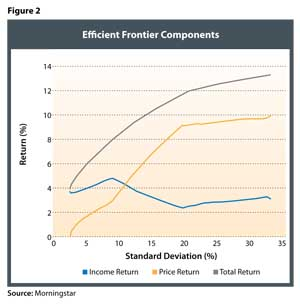The frontier in Figure 2 (the green line) is the traditional mean-variance efficient frontier that is created by maximizing total return and minimizing variance. The proportion of return generated by income is not specified. The income return (blue line) and price return (red line) are simply the component parts of the portfolio’s total return. If we change the definition of “optimal” to focus on income return1 only, we get a different efficient frontier, which lies below the total return efficient frontier, as depicted in Figure 3.Note that the income return frontier in figure 3 is truncated. It only extends as far as the highest yielding investment which, in this case, is US preferred stock. An investor seeking income greater than this amount (7.5 percent) would need to liquidate some of the portfolio (i.e., dip into the Price Return) to achieve a given consumption objective. However, we will focus on the differences in the portfolio allocations that fall within the same total return range and demonstrate the considerable differences that result from the different allocation methodologies.

The Income Return Utility Function

The traditional mean-variance utility function, as noted in equation one (below), is focused on maximising the total return for a given unit of risk: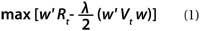Where:

• w = an N vector of asset class weights to be solved for
Rt = an N vector of total returns
λ = a aversion coefficient
Vt = an N by N total return covariance matrix

In order to determine the optimal income return portfolio we rewrite (1) to separate income from total return. We could, of course, separate income return from price return, but for an income-oriented investor, the total return should represent the “minimum-income frontier.” In other words, an income investor would never want less income than that generated by the total return frontier.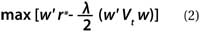For r*: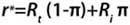Where:

• w = an N vector of asset class weights to be solved for
λ = a risk aversion coefficient
Vt = an N by N total return covariance matrix
Rt = an N vector of total returns
Ri = an N vector of income returns (yields)
π = preference for income return (yield) defined on the [0,1] interval

Critically, the risk component in the income return function (equation 2) is not based solely on income risk (i.e., the income return covariance matrix). Income investors without a fixed time horizon are very sensitive to total return risk which can be substantial. For example, while the standard deviation of the income return from small value has historically been relatively low, there is a significant amount of potential risk associated with the price return. The approach introduced in this paper, therefore, uses total return risk as the relevant risk measure. We also assume an income preference of 1 across the efficient frontier.

Estimating Total Return

For our analysis, we include the asset classes listed in Figure 4. Significantly, we don’t limit the opportunity set to higher-yielding asset classes since our goal is to strike a balance between total return, total risk, and income. The only arguably non-core asset class we include is preferred stock which, given the yield focus, cannot be ignored.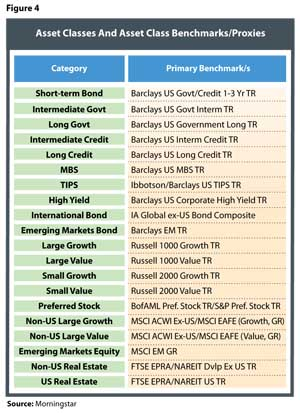To conform to standard practice, we use forward estimates of capital market returns. Predicting returns is a difficult exercise and can result in portfolios lacking in intuitive appeal and economic value. For example, Chopra and Ziemba (1993) estimate that at moderate risk tolerance levels, minimum variance optimisation is 11 times more sensitive to estimation error of returns than to estimation error in the covariance matrix. Our returns are based on the Sharpe-Lintner-Mossin-Treynor Capital Asset Pricing Model (CAPM). More specifically, we use the reverse optimisation procedure from Sharpe , which is also the starting point for the Black-Litterman model (see Black and Litterman ). The process is described in equation 3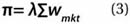Where:

• Π = an N vector of excess returns
λ = the risk aversion coefficient
= an N by N covariance matrix of excess returns
wmkt = an N vector of the market capitalization weights (the CAPM market portfolio)

Market capitalisation data are used to estimate the CAPM market portfolio. For most of our asset classes, we use index weights as a proxy for market weights. The market capitalisation for equities is based on data from the World Bank and market capitalisation for fixed income is based on data from the Bank for International Settlements.

The market capitalisation values for real estate are based on an approach used in Idzorek, Barad, and Meier , using J.P. Morgan Asset Management Real Estate’s estimate of the value of direct US real estate investment

The covariance matrix is constructed using monthly returns from January 1993 to April 2012. The assumed risk-free rate is 3.83 percent2 and the market risk premium is 4.35 percent, which was solved through reverse optimisation to create a large-cap domestic equity premium of 6.35 percent Relative market capitalisation weights and the resulting return estimates are included in Figure 5.

The values in Figure 5 are assumed to be the total return values for our analysis. We then split this total return into price and income returns. For fixed income, we assume that 100 percent of the total return comes from income and for equities we use asset class history to determine the income and price return split. For TIPS, which have their principal adjusted, we do the same.For equities, price appreciation is going to drive the majority of the return, except for preferred stocks, where the entire total return is assumed to be income return. With respect to dividends, yields are estimated based on recent dividend yields for the respective equity indices as well as long-term general historical trends. Covariances are calculated from the historical returns. The average return and standard deviation for the income and price return components of the total return are included in Figure 6. While the total return will always be the sum of the income return and price return, the standard deviations are not additive.

Figure 6 provides information on the how the relative efficiency of a portfolio (using minimum variance criteria) could vary, depending upon the investor’s underlying goal. For example, an investor entirely focused on income would likely consider long-dated government bonds an attractive investment since they offer a relatively high level of income return with a low standard deviation of income; however, they also have significant price return volatility. An investor purchasing a bond directly and planning to hold it to maturity would effectively experience no volatility. However, we assume the investor doesn’t have a fixed horizon and is thus sensitive to price movements.

For a larger view, please click on the image above.

Optimisation Results

In this section we explore the differences that result from optimising a portfolio using the traditional total return approach (based on equation 1) versus one in which we use an income approach (equation 2).

Figures 7 and 8 demonstrate the different portfolio allocations in an “area graph” format, based on income return and total return, respectively, for total returns ranging from 4.25 percent to 7.50 percent. The income return efficient frontiers were constructed using an approach that focuses on income return rather than total return, but the portfolios are contrasted from a total return perspective so that the reader can better understand the respective differences in the approaches, given the same overall target return.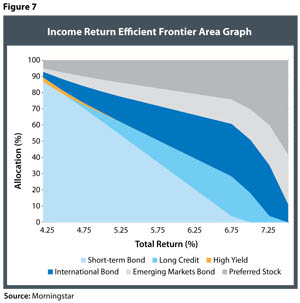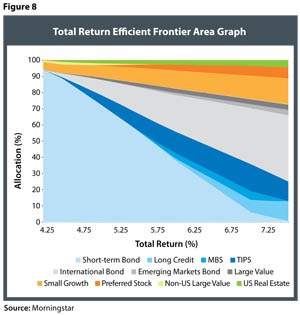Both optimisation approaches feature heavy allocations to short-term bonds for the lower return portfolios. However, there are considerable differences in the non-short-term portion. There are a number of asset classes that are featured prominently in the income return portfolios that do not appear in the total return portfolios (and vice versa). For example, the only equity component in the income return portfolios in Figure 7 is preferred stock, while the total return portfolios feature a number of different asset classes, such as large value, small growth, and US real estate. To give the reader a more precise example of the differences in the two approaches, Figure 9 has been included, which compares the optimal allocations for the income return and total return methodologies, given a total return of 6 percent.

The total return portfolios are more diversified than the income return portfolios. The likely reason for this is that the total return portfolios are focused on total return and are indifferent to the source of that return. In the income portfolios, we seek to maximise income while minimising total risk. This additional restriction limits the feasible set. The information in Figures 7 and 9 suggests that an investor seeking to build an income-efficient portfolio should consider including asset classes such as preferred stock, emerging market bonds, international bonds, long-term bonds, and (potentially) short-term bonds to create efficient portfolios. An investor looking to maximise income within a diversified portfolio framework could substitute short-term bonds with high-yield bonds (for example) to increase the yield.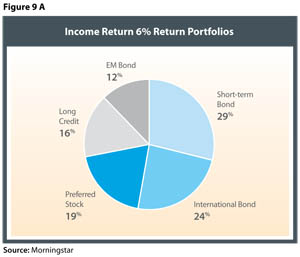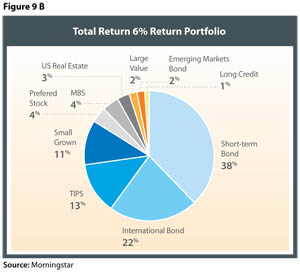A Word On Tax Efficiency

Although we have not incorporated taxes into our analysis, a word on tax efficiency is in order. A common critique of income-oriented investing is that it is tax-inefficient because income is taxed immediately, while capital return can be deferred. While this is important to note, potential tax liability becomes increasingly less important to an investor as the preference for consistent income increases. To illustrate this effect we use the following power utility function: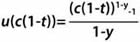Where:
C = income
γ = income risk aversion level
t = tax income rate

In Figure 10 we graph two utility curves, one assuming no taxes, the other assuming a 35 percent tax rate. We also show them at different levels of risk aversion, 2.5 (generally considered to be a “moderate” level of risk aversion and the comparatively high 5. Given that this sort of product is usually aimed at very risk-averse investors, we believe that 5 is reasonable.

For a larger view, please click on the image above.

Panels A and B in Figure 10 demonstrate that as the level of income increases, the post-tax utility and the pre-tax utility converge; albeit very slowly for a moderate risk investor. As risk aversion goes up, however, we see the two converge rapidly as the tax cost is outweighed by the benefit of income’s surety. It is trivial to show that as tax liability drops and risk aversion increases, the utility values converge with increasing rapidity. The economic take-away here may be that less wealthy investors in comparatively low income brackets with relatively little risk capacity (which we define as being embedded in the risk aversion coefficient) will derive the greatest utility from an income-oriented product.

Conclusions

As more investors approach retirement, they will increasingly seek solutions to help them generate income. Additionally, increasing levels of risk aversion due both to the life stage of these investors and concerns about increased market volatility are pushing them toward less risky options. Despite the potential appeal of income-focused products, few diversified options currently exist to meet this objective. This is likely due to the industry’s focus on total return.

This paper introduced a new framework to determine how to build an asset allocation with an income focus. Portfolios focused on income are likely use asset classes that may be ignored in traditional optimisations, such as preferred stock, long-dated bonds, and emerging market bonds. Income-efficient portfolios may have asset allocations that vary considerably from traditional mean variance portfolios (focused on total returns) and therefore investors seeking income are likely best served using approaches or portfolios built with this goal in mind.

Endnotes And References

1. Based on Equation 2, which is introduced later in the paper
2. This is based on 24 month average yield of long-term Treasury bonds, i.e., uses a long-term proxy as the risk-free rate, versus something shorter term in nature (e.g., 3 month Treasury bills)
• Black, Fischer, and Robert Litterman. (1992). “Global Portfolio Optimization.” Financial Analysts Journal, September/October, 28-43.
• Cochrane, John H. 2001. Asset Pricing. Princeton University Press
• Chopra, V. K. and W. T. Ziemba. (1993). “The Effect of Errors in Means, Variances, and Covariances on Optimal Portfolio Choice”, Journal of Portfolio Management, Winter, 6–11.
• Idzorek, Thomas, Mike Barad, and Steve Meier. (2007). “Global Commercial Real Estate.” The Journal of Portfolio Management. Special Real Estate Issue, 37 – 53.
• Sharpe, William F. (1974). “Imputing Expected Security Returns from Portfolio Composition.” Journal of Financial and Quantitative Analysis, June, 463-472.